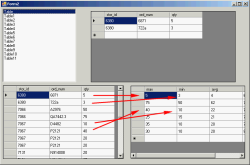# SQL Server 2005 Training, Day 1, Part 2

## SQL Server 2005 Training, Day 1, Part 2

This part contents mostly concerns coding on VB.NET which will interacts with SQL server on pubs database. The sample database can be download at SQL2000SampleDb. You do not need to complete Part I before.

### Letâ€™s start

1. #### Define a connection string module

Create new VB.NET Windows Application project and add new module to the project for add a connection string to SQL server. You need to alter this string to match your environment and keep database pointing to pubs.

```Module Module1 Public Sqlcon As String = "Server=BKKSQL2005;uid=sa;password=123456;database=pubs" End Module```

3. #### Query table sales by year

Design form1.vb

• Back to form1.vb.
• Add new Textbox as TextBox1, TextBox2, TextBox3, TextBox4 and TextBox5 to the form.
• Add new Button as Button1 and Button2 to the form.
• Add DataGridView to the form.

Coding form1.vb

• Add code below on this form.
• `Imports System.Data.SqlClient`
• Double click Button1 and put below code into it.
• ``` Dim strsql As String = "select title_id " For i As Integer = CInt(TextBox1.Text) To CInt(TextBox2.Text) strsql &= ", sum(case year(ord_date) when " & i & " then qty else 0 end) as Y" & i Next strsql &= " from sales group by title_id with rollup"   Dim DA As New SqlDataAdapter(strsql, Module1.Sqlcon) Dim DT As New DataTable DA.Fill(DT)   Dim str1 As String = "" For j As Integer = 1 To DT.Columns.Count - 1 Dim dc As DataColumn = DT.Columns(j) If str1 <> "" Then str1 = str1 & "+" str1 = str1 & dc.ColumnName Next   DT.Columns.Add("Sum1", GetType(Integer), str1)   Dim dr As DataRow = DT.Rows(DT.Rows.Count - 1) dr(0) = "Total" DataGridView1.DataSource = DT```
• Try compile and run the project, enter ‘1992’ on TextBox1 as start year and ‘1994’ on TextBox2 as end year and click the ‘View by Year’ button. Youâ€™ll see the result as below.
•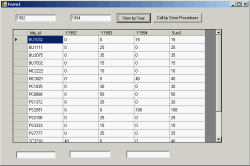5. #### Call a store procedure

This example will modify code from previous example and you need a store procedure â€˜test_aâ€™ which can be found in Part I or download sql to create a procedure at here – test_a.sql

There are 3 different coding styles that will give the same result for calling store procedure. The example will try to call a store procedure â€˜test_aâ€™ which receive two inputs (start year and end year).

From previous Form1, modify Button1_Click event to be one of these code.

1. Sending parameters along with execute string.
``` Dim strsql As String = "exec test_a '" & TextBox1.Text & "', '" & TextBox2.Text & "'" Dim DA As New SqlDataAdapter(strsql, Module1.Sqlcon) Dim DT As New DataTable DA.Fill(DT) DataGridView1.DataSource = DT```
2. Sending parameters by adding as SqlParameters.
``` Dim cn As New SqlConnection(Module1.Sqlcon) Dim cmd As New SqlCommand("test_a", cn) cmd.CommandType = CommandType.StoredProcedure Dim P1 As SqlParameter = cmd.Parameters.Add("@Year1", SqlDbType.NVarChar) P1.Value = TextBox1.Text P1 = cmd.Parameters.Add("@Year2", SqlDbType.NVarChar) P1.Value = TextBox2.Text   Dim DA As New SqlDataAdapter(cmd) Dim dt As New DataTable DA.Fill(dt) DataGridView1.DataSource = dt```
3. Using OleDB
```Imports System.Data.OleDb . . Dim cn As New OleDbConnection("Provider=SQLOLEDB;" & Module1.Sqlcon) Dim cmd As New OleDbCommand("{call test_a (?,?)}", cn) Dim P1 As OleDbParameter = cmd.Parameters.Add("@Year1", OleDbType.VarWChar) P1.Value = TextBox1.Text P1 = cmd.Parameters.Add("@Year2", OleDbType.VarWChar) P1.Value = TextBox2.Text Dim DA As New OleDbDataAdapter(cmd) Dim dt As New DataTable DA.Fill(dt) DataGridView1.DataSource = dt```

The result will look like previous example. You can download the modified source code at here – TrainingSQL2.zip.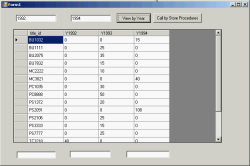7. #### Get return value from store procedure

There are 3 different coding styles as like in previous example. Modify source in Form1.Button1_Click method with the following code again.

1. Sending parameters along with execute string
``` Dim strsql As String = "declare @i int exec @i = test_a '" & TextBox1.Text & "', '" & TextBox2.Text & "' select @i as num1" Dim DA As New SqlDataAdapter(strsql, Module1.Sqlcon) Dim DS As New DataSet DA.Fill(DS) DataGridView1.DataSource = DS.Tables(0) Dim i As Integer = DS.Tables(1).Rows(0)("num1") MsgBox(i)```
2. Sending parameters by using SqlParameter
``` Dim cn As New SqlConnection(Module1.Sqlcon) Dim cmd As New SqlCommand("test_a", cn) cmd.CommandType = CommandType.StoredProcedure Dim P2 As SqlParameter = cmd.Parameters.Add("@Return", SqlDbType.Int) P2.Direction = ParameterDirection.ReturnValue Dim P1 As SqlParameter = cmd.Parameters.Add("@Year1", SqlDbType.NVarChar) P1.Value = TextBox1.Text P1 = cmd.Parameters.Add("@Year2", SqlDbType.NVarChar) P1.Value = TextBox2.Text Dim DA As New SqlDataAdapter(cmd) Dim dt As New DataTable DA.Fill(dt) DataGridView1.DataSource = dt MsgBox(P2.Value)```
3. Using OleDB
``` Dim cn As New OleDbConnection("Provider=SQLOLEDB;" & Module1.Sqlcon) Dim cmd As New OleDbCommand("{? = call test_a (?,?)}", cn) Dim P2 As OleDbParameter = cmd.Parameters.Add("@Return", OleDbType.Integer) P2.Direction = ParameterDirection.ReturnValue Dim P1 As OleDbParameter = cmd.Parameters.Add("@Year1", OleDbType.VarWChar) P1.Value = TextBox1.Text P1 = cmd.Parameters.Add("@Year2", OleDbType.VarWChar) P1.Value = TextBox2.Text Dim DA As New OleDbDataAdapter(cmd) Dim dt As New DataTable DA.Fill(dt) DataGridView1.DataSource = dt MsgBox(P2.Value)```

The result will look like previous example but add a pop-up message box the return value from the store procedure. You can download the source code at here – TrainingSQL3.zip.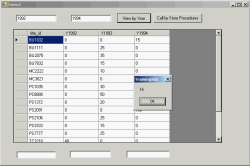9. #### Get return values from store procedure

This example, you need a store procedure â€˜test_a2â€™ which can be found here – test_a2.sql. This store procedure receives two inputs as the store procedure ‘test_a’ but it returns more additional values which are @sum1, @avg1 and a return value.
There are 3 different coding styles as like in previous example. Modify source in Form1.Button1_Click method with the following code again. The additional values that are returned from the store procedure will be place into TextBox3, TextBox4 and TextBox5.

1. Sending parameters along with execute string
``` Dim strsql As String = "declare @i int,@j int, @k int exec @i = test_a2 '" & TextBox1.Text & "', '" & TextBox2.Text & "',@j out, @k out select @i as num1,@j,@k" Dim DA As New SqlDataAdapter(strsql, Module1.Sqlcon) Dim DS As New DataSet DA.Fill(DS) DataGridView1.DataSource = DS.Tables(0) Dim dr As DataRow = DS.Tables(1).Rows(0) TextBox3.Text = dr(0) TextBox4.Text = dr(1) TextBox5.Text = dr(2)```
2. Sending parameters by using SqlParameter
Note: The parameter’s name must match as in the store procedure.

``` Dim cn As New SqlConnection(Module1.Sqlcon) Dim cmd As New SqlCommand("test_a2", cn) cmd.CommandType = CommandType.StoredProcedure Dim P2 As SqlParameter = cmd.Parameters.Add("@Return", SqlDbType.Int) P2.Direction = ParameterDirection.ReturnValue Dim P1 As SqlParameter = cmd.Parameters.Add("@Year1", SqlDbType.NVarChar) P1.Value = TextBox1.Text P1 = cmd.Parameters.Add("@Year2", SqlDbType.NVarChar) P1.Value = TextBox2.Text Dim P3 As SqlParameter = cmd.Parameters.Add("@sum1", SqlDbType.Int) P3.Direction = ParameterDirection.Output Dim P4 As SqlParameter = cmd.Parameters.Add("@avg1", SqlDbType.Int) P4.Direction = ParameterDirection.Output Dim DA As New SqlDataAdapter(cmd) Dim dt As New DataTable DA.Fill(dt) DataGridView1.DataSource = dt TextBox3.Text = P2.Value TextBox4.Text = P3.Value TextBox5.Text = P4.Value```
3. Using OleDB
``` Dim cn As New OleDbConnection("Provider=SQLOLEDB;" & Module1.Sqlcon) Dim cmd As New OleDbCommand("{? = call test_a2 (?,?,?,?)}", cn) Dim P2 As OleDbParameter = cmd.Parameters.Add("@Return", OleDbType.Integer) P2.Direction = ParameterDirection.ReturnValue Dim P1 As OleDbParameter = cmd.Parameters.Add("@Year1", OleDbType.VarWChar) P1.Value = TextBox1.Text P1 = cmd.Parameters.Add("@Year2", OleDbType.VarWChar) P1.Value = TextBox2.Text Dim P3 As OleDbParameter = cmd.Parameters.Add("@sum1", OleDbType.Integer) P3.Direction = ParameterDirection.Output Dim P4 As OleDbParameter = cmd.Parameters.Add("@avg1", OleDbType.Integer) P4.Direction = ParameterDirection.Output Dim DA As New OleDbDataAdapter(cmd) Dim dt As New DataTable DA.Fill(dt) DataGridView1.DataSource = dt TextBox3.Text = P2.Value TextBox4.Text = P3.Value TextBox5.Text = P4.Value```

The result will look like previous example but add a pop-up message box the return value from the store procedure. You can download the source code at here – TrainingSQL4.zip.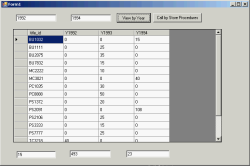11. #### Using max, min, and average

This example shows how to using function max, min and average by compute from qty field on sales table.

Design form2.vb

• Add new form on the project.
• Add new ListBox1 to the form.
• Add DataGridView1, DataGridView2 and DataGridView3 to the form.

Coding form2.vb
Add below code to the form.

```Imports System.Data.SqlClient   Public Class Form2 Dim ds As New DataSet Private Sub Form2_Load(ByVal sender As System.Object, ByVal e As System.EventArgs) Handles MyBase.Load Dim strsql As String = "select stor_id,ord_num, qty from sales order by stor_id " & _ " compute max(qty),min(qty), avg(qty) by stor_id" Dim da As New SqlDataAdapter(strsql, Module1.Sqlcon)   da.Fill(ds) 'MsgBox(ds.Tables.Count) For Each dt As DataTable In ds.Tables ListBox1.Items.Add(dt.TableName) Next Dim dt3 As DataTable = ds.Tables(0).Clone Dim dt4 As DataTable = ds.Tables(1).Clone dt4.Columns.Add("Stor_id", GetType(Integer)) For i As Integer = 0 To ds.Tables.Count - 1 Step 2 Dim dt As DataTable = ds.Tables(i) Dim Stor_ID As Integer = dt.Rows(0)("stor_id") For Each dr As DataRow In dt.Rows 'dt3.ImportRow(dr) Dim dr2 As DataRow = dt3.NewRow dr2.ItemArray = dr.ItemArray dt3.Rows.Add(dr2) Next   Dim dr5 As DataRow = ds.Tables(i + 1).Rows(0) dt4.ImportRow(dr5) Dim dr4 As DataRow = dt4.Rows(dt4.Rows.Count - 1) dr4("stor_id") = Stor_ID   Next DataGridView2.DataSource = dt3 DataGridView3.DataSource = dt4     End Sub   Private Sub ListBox1_SelectedIndexChanged(ByVal sender As System.Object, ByVal e As System.EventArgs) Handles ListBox1.SelectedIndexChanged Dim DT As DataTable DT = ds.Tables(ListBox1.Text) DataGridView1.DataSource = DT End Sub End Class```

Change startup form on the project by right-click on the project’s name and select properties and change startup form from ‘Form1’ to ‘Form2’. Compile and run the project.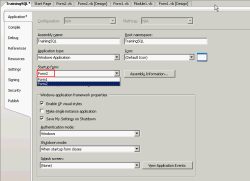You’ll see that on DataGridView1 will be changed when you change value on ListBox1. On DataGridView2 shows result from sql query command and DataGridView3 shows computed values (max, min and average) of each stor_id. You can download the source code at here – TrainingSQL5.zip.